### Discover more content...

Enter some keywords in the search box above, we will do our best to offer you relevant results.

### We're sorry!

We couldn't find any results for your search. Please try again with another keywords.

## 一. Motivation

1. 学习性能下降，知识越多，吸收知识（输入），并且精通知识（学习）的速度就越慢。
2. 过多的特征难于分辨，你很难第一时间认识某个特征代表的意义。
3. 特征冗余，如下图所示，厘米和英尺就是一对冗余特征，他们本身代表的意义是一样的，并且能够相互转换。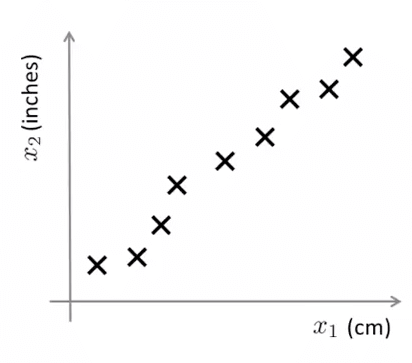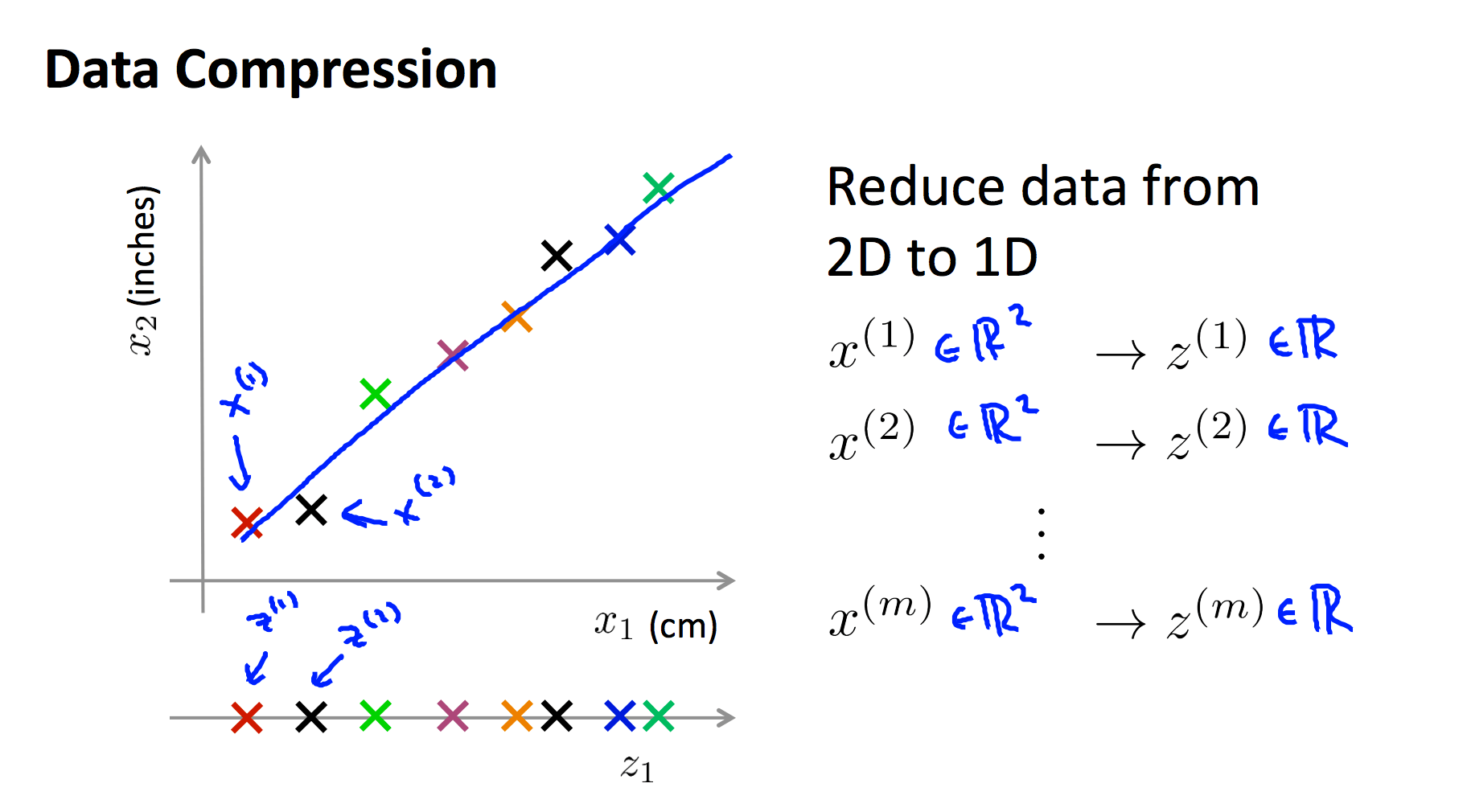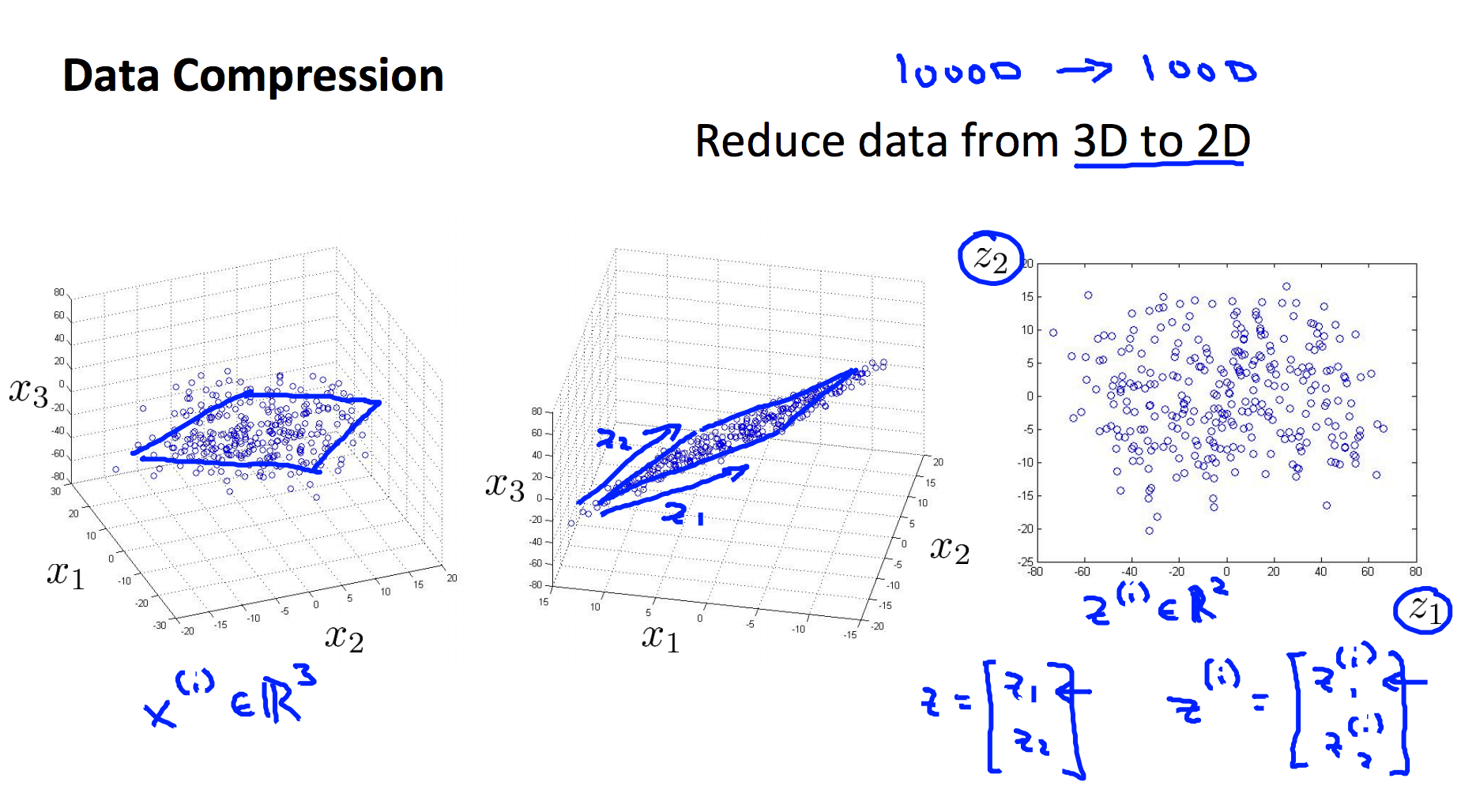1. 压缩数据
2. 数据可视化

## 二. Principal Component Analysis 主成分分析

PCA，Principle Component Analysis，即主成分分析法，是特征降维的最常用手段。顾名思义，PCA 能从冗余特征中提取主要成分，在不太损失模型质量的情况下，提升了模型训练速度。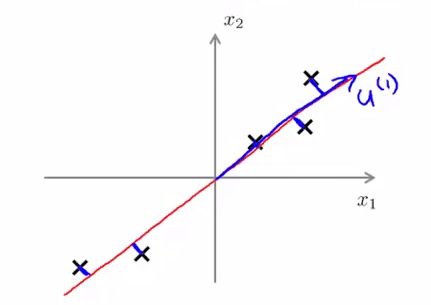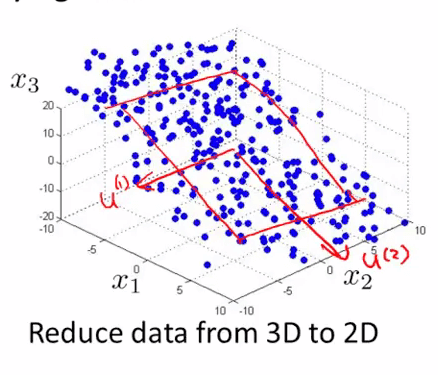### 1. 区别

PCA 和 线性回归的区别是：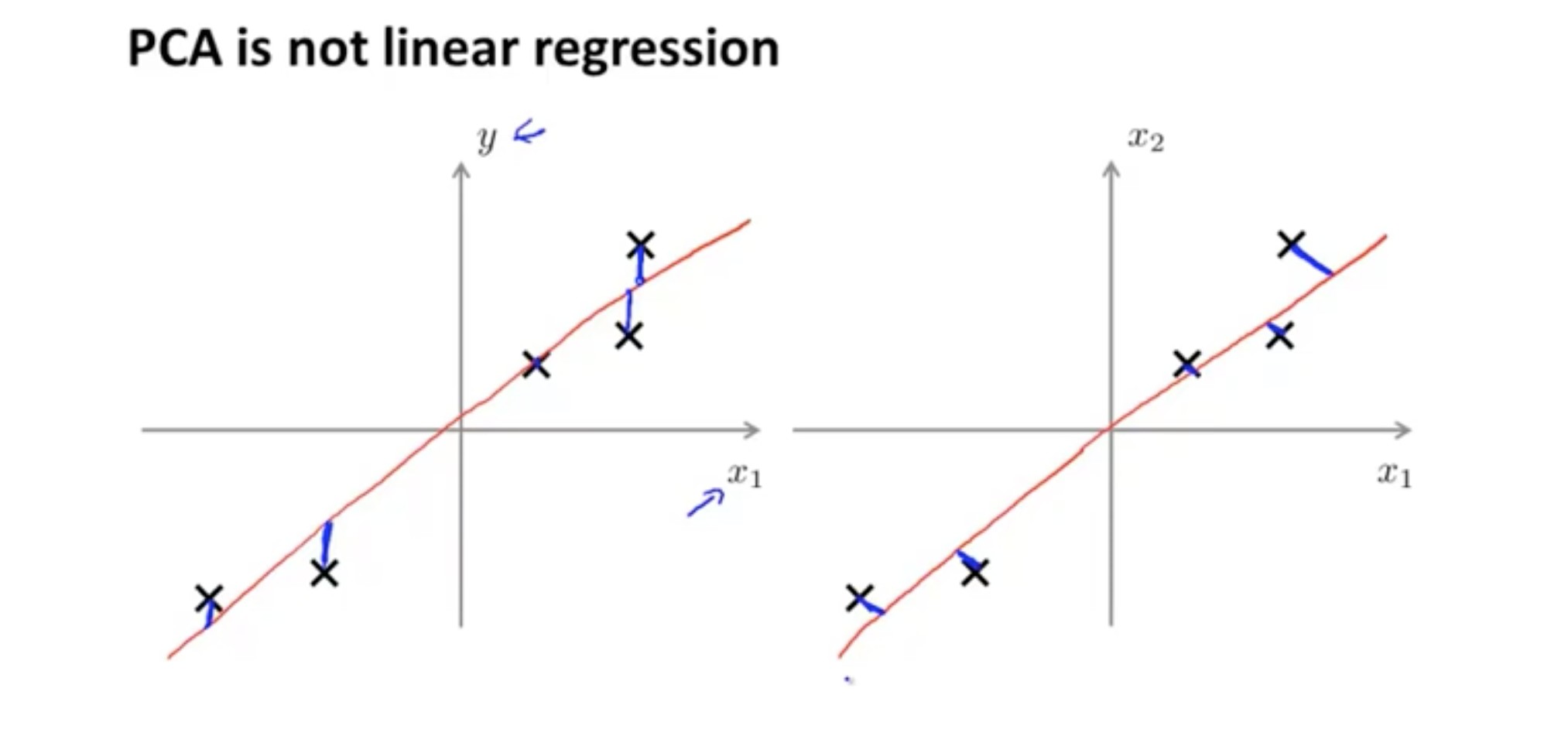### 2. 算法流程

$$x^{(i)}_j=\frac{x^{(i)}_j-\mu_j}{s_j}$$

$\mu_j$ 为特征 j 的均值，sj 为特征 j 的标准差。

$$\Sigma =\frac{1}{m}\sum_{i=1}{m}(x^{(i)})(x^{(i)})^T=\frac{1}{m} \cdot X^TX$$

$$(U,S,V^T)=SVD(\Sigma )$$

$$U_{reduce}=(\mu^{(1)},\mu^{(2)},\cdots,\mu^{(k)})$$

$$z^{(i)}=U^{T}_{reduce} \cdot x^{(i)}$$

### 3. 特征还原

$$z=U^T_{reduce}x$$

$$x_{approx}=U_{reduce}z$$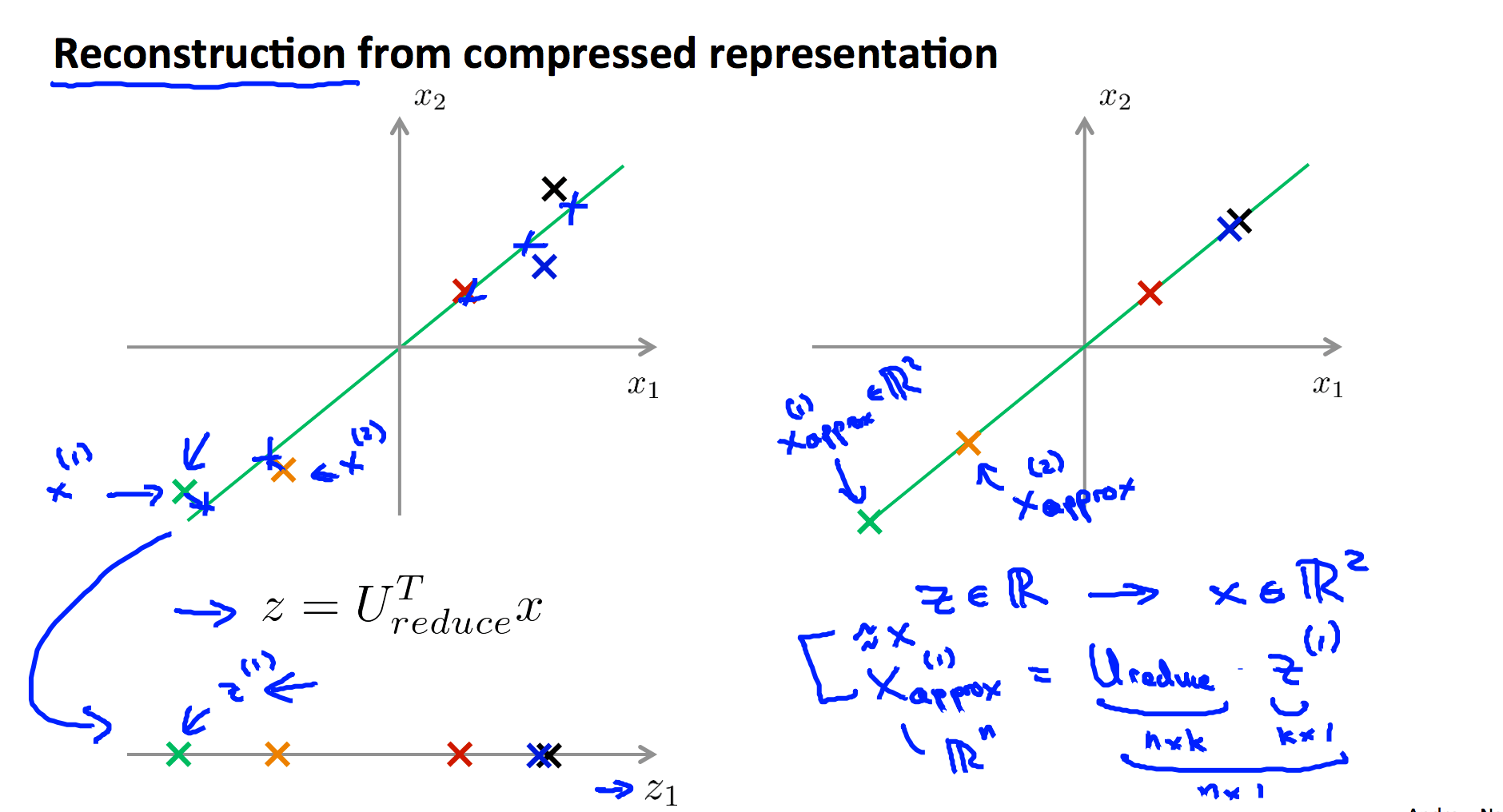### 4. 降维多少才合适？

$$\min \frac{1}{m}\sum_{j=1}^{m}\left \| x^{(i)}-x^{(i)}_{approx} \right \|^2$$

$$\frac{1}{m}\sum_{j=1}^{m}\left \| x^{(i)} \right \|^2$$

$$\frac{\min \frac{1}{m}\sum_{j=1}^{m}\left \| x^{(i)}-x^{(i)}_{approx} \right \|^2}{\frac{1}{m}\sum_{j=1}^{m}\left \| x^{(i)} \right \|^2} \leqslant \epsilon$$

### 5. 不要提前优化

PCA 用来解决过拟合的问题是错误的选择，请考虑正则化的方式解决过拟合的问题

PCA通常都是 被用来 压缩数据的 以减少内存使用 或硬盘空间占用 或者用来可视化数据

## 三. Principal Component Analysis 测试

### 1. Question 1

Consider the following 2D dataset: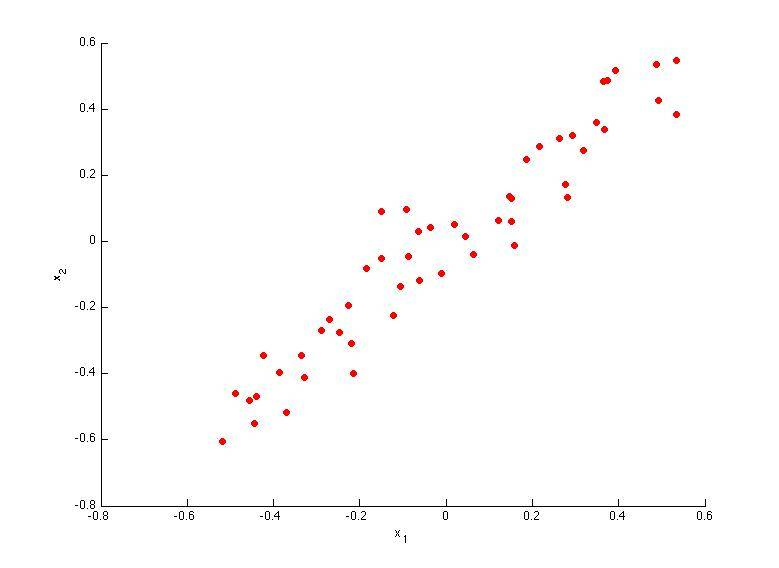Which of the following figures correspond to possible values that PCA may return for u(1) (the first eigenvector / first principal component)? Check all that apply (you may have to check more than one figure).

A.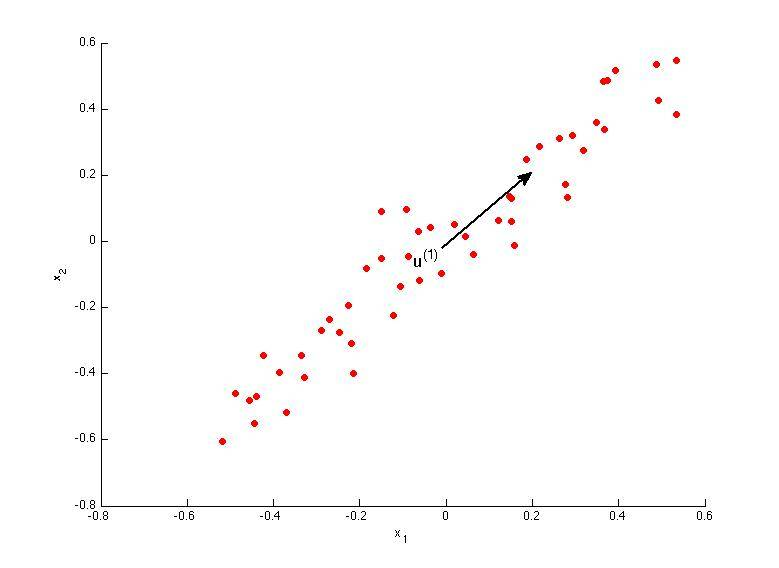B.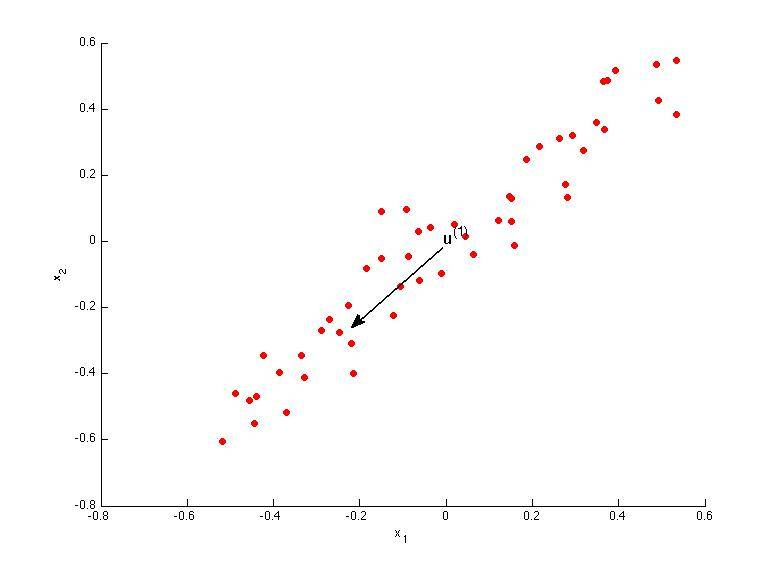C.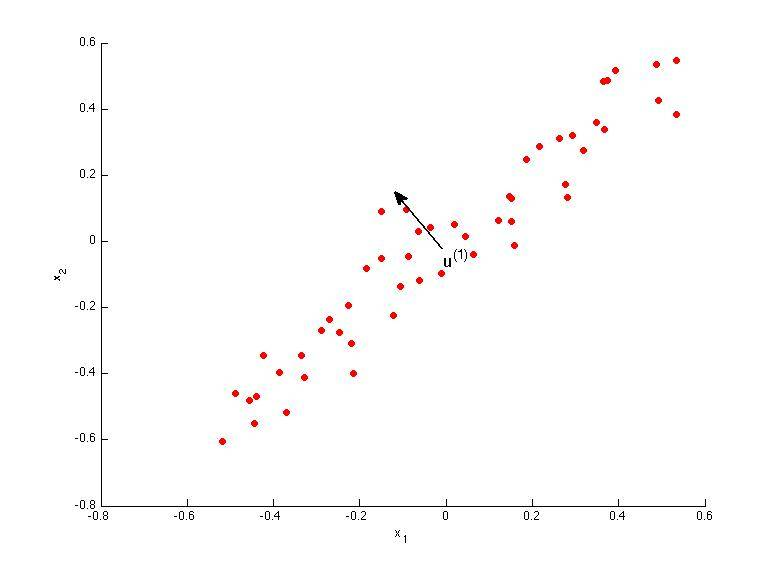D.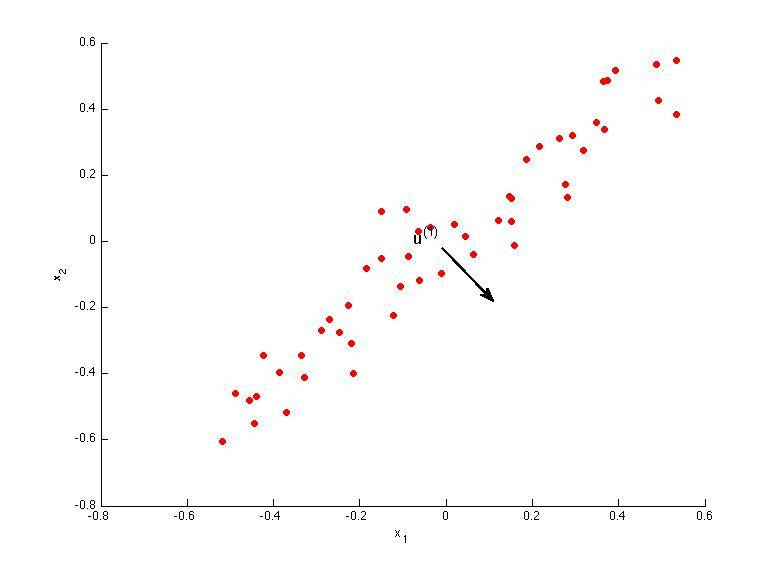### 2. Question 2

Which of the following is a reasonable way to select the number of principal components k?

(Recall that n is the dimensionality of the input data and m is the number of input examples.)

A. Choose k to be the smallest value so that at least 1% of the variance is retained.

B. Choose k to be the smallest value so that at least 99% of the variance is retained.

C. Choose the value of k that minimizes the approximation error $\frac{1}{m}\sum^{m}_{i=1}\left \| x^{(i)} - x_{approx}^{(i)} \right \|^{2}$.

D. Choose k to be 99% of n (i.e., k=0.99∗n, rounded to the nearest integer).

### 3. Question 3

Suppose someone tells you that they ran PCA in such a way that "95% of the variance was retained." What is an equivalent statement to this?

A.

$$\frac{\frac{1}{m}\sum^{m}_{i=1}\left \| x^{(i)} \right \|^{2}}{\frac{1}{m}\sum^{m}_{i=1}\left \| x^{(i)} - x_{approx}^{(i)} \right \|^{2}} \geqslant 0.05$$

B.

$$\frac{\frac{1}{m}\sum^{m}_{i=1}\left \| x^{(i)} \right \|^{2}}{\frac{1}{m}\sum^{m}_{i=1}\left \| x^{(i)} - x_{approx}^{(i)} \right \|^{2}} \leqslant 0.95$$

C.

$$\frac{\frac{1}{m}\sum^{m}_{i=1}\left \| x^{(i)} - x_{approx}^{(i)} \right \|^{2}}{\frac{1}{m}\sum^{m}_{i=1}\left \| x^{(i)} \right \|^{2}} \leqslant 0.05$$

D.

$$\frac{\frac{1}{m}\sum^{m}_{i=1}\left \| x^{(i)} \right \|^{2}}{\frac{1}{m}\sum^{m}_{i=1}\left \| x^{(i)} - x_{approx}^{(i)} \right \|^{2}} \leqslant 0.05$$

### 4. Question 4

Which of the following statements are true? Check all that apply.

A. If the input features are on very different scales, it is a good idea to perform feature scaling before applying PCA.

B. Feature scaling is not useful for PCA, since the eigenvector calculation (such as using Octave's svd(Sigma) routine) takes care of this automatically.

C. Given an input $x \in \mathbb{R}^{n}$, PCA compresses it to a lower-dimensional vector $z \in \mathbb{R}^{k}$.

D. PCA can be used only to reduce the dimensionality of data by 1 (such as 3D to 2D, or 2D to 1D).

### 5. Question 5

Which of the following are recommended applications of PCA? Select all that apply.

A. To get more features to feed into a learning algorithm.

B. Data compression: Reduce the dimension of your data, so that it takes up less memory / disk space.

C. Data visualization: Reduce data to 2D (or 3D) so that it can be plotted.

D. Data compression: Reduce the dimension of your input data $x^{(i)}$, which will be used in a supervised learning algorithm (i.e., use PCA so that your supervised learning algorithm runs faster).

GitHub Repo：Halfrost-Field

Follow: halfrost · GitHubPrevious Post

Next Post